# Scientific Problem-Solving: DMAIC in Terms of Y = f(x)

By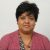Aditi Gilman

A major advantage provided by the implementation of Six Sigma is changing the thought process from trial and error to Y = f(x). Most training programs for Six Sigma seem to focus on teaching the tools rather than driving home the importance of scientific problem-solving for process improvement. That is where breakthroughs really begin. One of the best ways to transform an organization from one using firefighting techniques to one thinking in terms of Y = f(x), is to start in the classroom when Six Sigma techniques are first taught.

### Defining Six Sigma Success

Six Sigma methodologies are used to translate black box problems into Y = f(x) using statistical analysis as demonstrated in Figure 1. A Six Sigma project team aims to solve a problem through identification of the true root cause and application of a solution that reduces the impact of the root cause. The turning point of the project is when the solution becomes transparent, and the output (Y) can be consistently predicted using the Y = f(x) relationship as validated by the DMAIC methodology. Most literature available on Six Sigma today fails to emphasize this turning point.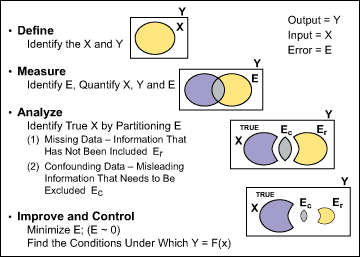Figure 1: A Scientific Model of DMAIC

A Six Sigma project is successful when the team is able to solve Y = f(x).That is, relate all the key inputs to each expected output within a small magnitude of error (Figure 2).

Handpicked Content:   Six Sigma: PDCA on Steroids?

Y = Sy = Sx + Se(ignoring Se where e is negligible)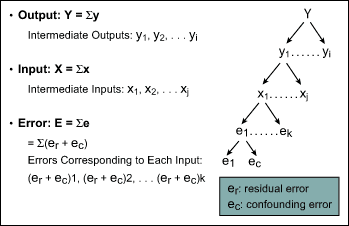Figure 2: Six Sigma Project Model

### DMAIC: Revealing the Predictive Power of Six Sigma

The DMAIC roadmap (Figure 3) shows the relationship between the Y and x factors. In the Define phase, the process is described qualitatively in relation to the business, using tools such as a SIPOC diagram. Expectations from the process are specified quantitatively through capture of the voice of the customer. The key outputs (Y) and critical-to-satisfaction elements are revealed. A detailed process map focused on the key outputs will provide a list of inputs. The Define phase identifies the key outputs and all the known inputs.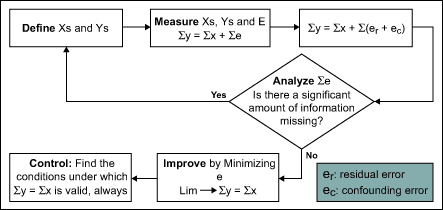Figure 3: DMAIC Roadmap

(The deliverables of each phase of a Six Sigma project as suggested in Figure 1 are slightly different from that proposed in most Six Sigma literature. Furthermore, Y = f(x) is a transfer function, but Figures 2 and 3 assume for the sake of simplicity that the mathematical operator is addition. The correct mathematical operator is revealed when the Y= f (x)is modeled [regression] in the Analyze phase.)

In the Measure phase, the list of x and Y variables are measured, including all possible error (E) components. This is where Lean thinking really meets Six Sigma. A thorough value stream mapping exercise will reveal the waste or errors as well as sources of variation in the process. When the error components are measured and arranged in the form of Y = f(x), the problem is restated in a hypothesis format, Ho: Y = f(X) vs. Ha: Y does not = f(X). Now the problem is in a form that lends itself to statistical analysis Figure 4).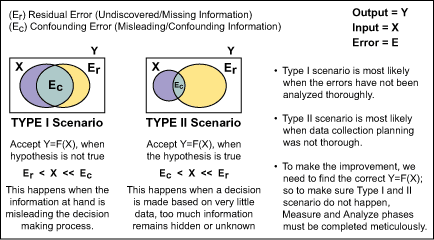Figure 4: Type I and Type II Errors

After analyzing the error components, if a significant amount of information is missing (residual error) then the Define and Measure phases must be revisited. That is, if Ha cannot be rejected then Ho must be re-formed. If Ha can be rejected, then the process-inherent error components are ready for further analysis in preparation for the Improve phase.

Handpicked Content:   Y = f(x) Roadmap: Telling the DMAIC Story Using Xs and Ys

To improve the process, concentrate all efforts on relating the sources of variation to the identified waste imbedded in the process. A process map and information gathered during the Measure phase are helpful at this stage. Using Lean principles such as “pull” and “perfection” and the theory of constraints combined with design of experiments (DOE) and various improvement tools, a reduction or even elimination of process errors is achievable. Breakthrough improvement happens when the project team is able to bring the net E to zero and can simulate the output based on the inputs. How accurately the output is predicted is one measure of Six Sigma success.

Once the Improve phase has established how to predict the Y, the Control phase begins. During the Control phase, the conditions are determined under which the Y = f(x) relationship is always applicable. This is accomplished by performing DOE to validate the relationship. Understanding the impact of risks through completion of a failure modes and effects analysis of the improved state also is key. The final step is to assimilate the knowledge gained through the improvement process into the current system by implementing any necessary changes in procedure or statistical process control charts.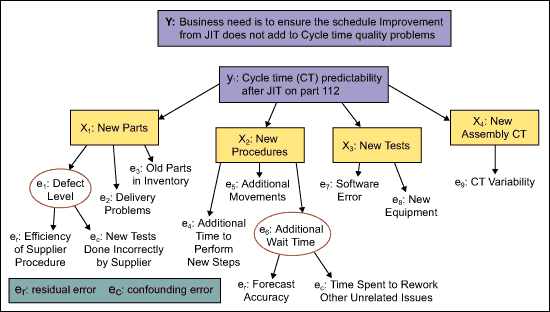Figure 5: Example Application of Six Sigma Scientific Thinking

With a top-down strategy, a balanced scorecard is often used to monitor lagging indicators (Ys) in terms of lead indicators (x’s). A bottom-up or rapid improvement using Six Sigma will often deploy Lean principles to perfect the process by removing all waste (error components). The Six Sigma methodology is successful as long as the organization learns to solve problems using the scientific method.

1.2.